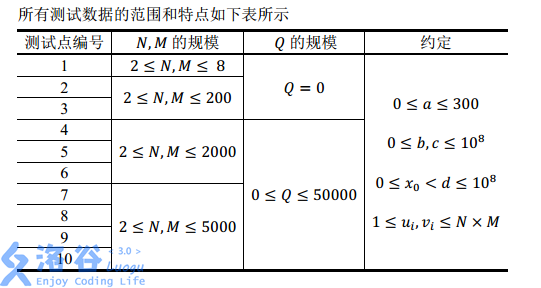P2354 [NOI2014]随机数生成器

• 322通过
• 1.1K提交
• 题目提供者
• 评测方式 云端评测
• 标签 模拟 贪心 NOI系列 2014 高性能
• 难度 省选/NOI-
• 时空限制 1000ms-5000ms / 256MB
• 提示：收藏到任务计划后，可在首页查看。

题目描述

小 H 最近在研究随机算法。随机算法往往需要通过调用随机数生成函数（例如 Pascal 中的 random 和 C/C++中的 rand）来获得随机性。事实上，随机数生成函数也并不是真正的“随机”，其一般都是利用某个算法计算得来的。

比如，下面这个二次多项式递推算法就是一个常用算法：

算法选定非负整数 $x_0,a,b,c,d$ 作为随机种子，并采用如下递推公式进行计算。

对于任意 $i ≥ 1,x_i=(a \times x_{i-1}^2+b \times x_{i-1}+c)\mod d$ 这样可以得到一个任意长度的非负整数数列$\{x_i\},i \ge 1$，一般来说，我们认为这个数列是随机的。

利用随机序列 ${xi},i≥1$，我们还可以采用如下算法来产生一个 $1$ 到 $K$ 的随机排列$\{ Ti \},i=1 ... k$：

1、初始设 $T$ 为 $1$ 到 $K$ 的递增序列；

2、对 $T$ 进行 $K$ 次交换，第 $i$ 次交换，交换 $T_i$ 和 $T_{x_i \mod i + 1}$ 的值。

此外，小 H 在这 $K$ 次交换的基础上，又额外进行了 $Q$ 次交换操作，对于第i 次额外交换，小 H 会选定两个下标 $u_i$ 和 $v_i$，并交换 $T_{u_i}$ 和 $T_{v_i}$ 的值。

为了检验这个随机排列生成算法的实用性，小 H 设计了如下问题：

小 H 有一个 $N$ 行 $M$ 列的棋盘，她首先按照上述过程，通过 $N \times M + Q$ 次交换操作，生成了一个 $1\sim N \times M$ 的随机排列 $\{Ti\},i=1 ... N \times M$，然后将这 $N \times M$ 个数逐行逐列依次填入这个棋盘：也就是第 $i$ 行第 $j$ 列的格子上所填入的数应为 $T_{(i-1) \times M+u_j}$。

接着小 H 希望从棋盘的左上角，也就是第一行第一列的格子出发，每次向右走或者向下走，在不走出棋盘的前提下，走到棋盘的右下角，也就是第 $N$ 行第 $M$ 列的格子。

小 H 把所经过格子上的数字都记录了下来，并从小到大排序，这样，对于任何一条合法的移动路径，小 H 都可以得到一个长度为 $N + M - 1$ 的升序序列，我们称之为路径序列。

小 H 想知道，她可能得到的字典序最小的路径序列应该是怎样的呢？

输入输出格式

输入格式：

第1行包含5个整数，依次为 $x_0,a,b,c,d$ ，描述小H采用的随机数生成算法所需的随机种子。

第2行包含三个整数 $N,M,Q$ ，表示小H希望生成一个1到 $N \times M$ 的排列来填入她 $N$ 行 $M$ 列的棋盘，并且小H在初始的 $N \times M$ 次交换操作后，又进行了 $Q$ 次额外的交换操作。

接下来 $Q$ 行，第 $i$ 行包含两个整数 $u_i,v_i$，表示第 $i$ 次额外交换操作将交换 $T_{u_i}$和 $T_{v_i}$ 的值。

输出格式：

输出一行，包含 $N+M-1$ 个由空格隔开的正整数，表示可以得到的字典序最小的路径序列。

输入输出样例

输入样例#1： 复制
1 3 5 1 71
3 4 3
1 7
9 9
4 9 
输出样例#1： 复制
1 2 6 8 9 12
输入样例#2： 复制
654321 209 111 23 70000001
10 10 0 
输出样例#2： 复制
1 3 7 10 14 15 16 21 23 30 44 52 55 70 72 88 94 95 97
输入样例#3： 复制
123456 137 701 101 10000007
20 20 0 
输出样例#3： 复制
1 10 12 14 16 26 32 38 44 46 61 81 84 101 126 128 135 140 152 156 201 206 237 242 243 253 259 269 278 279 291 298 338 345 347 352 354 383 395


说明

对于样例 1，根据输入的随机种子，小 H 所得到的前 12 个随机数xi为：

9 5 30 11 64 42 36 22 1 9 5 30

根据这 12 个随机数，小 H 在进行初始的 12 次交换操作后得到的排列为：

6 9 1 4 5 11 12 2 7 10 3 8

在进行额外的 3 次交换操作之后，小 H 得到的最终的随机排列为：

12 9 1 7 5 11 6 2 4 10 3 8

12 9 1 7
5 11 6 2
4 10 3 8

最优路径依次经过的数字为 ：12-9-1-6-28。提示
标程仅供做题后或实在无思路时参考。
请自觉、自律地使用该功能并请对自己的学习负责。
如果发现恶意抄袭标程，将按照I类违反进行处理。